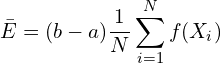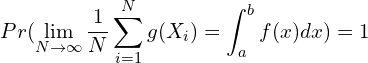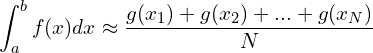# 使用蒙特卡洛计算定积分（附Python代码）

### 2. 蒙特卡洛求定积分：期望法"""
This code is supported by the website: https://www.guanjihuan.com
The newest version of this code is on the web page: https://www.guanjihuan.com/archives/1145
"""

import numpy as np
import random
import time

def integral():   # 直接数值积分
integral_value = 0
for x in np.arange(0, 1, 1/10**7):
integral_value = integral_value + x**2*(1/10**7)   # 对x^2在0和1之间积分
return integral_value

def MC_1():  # 蒙特卡洛求定积分1：投点法
n = 10**7
x_min, x_max = 0.0, 1.0
y_min, y_max = 0.0, 1.0
count = 0
for i in range(0, n):
x = random.uniform(x_min, x_max)
y = random.uniform(y_min, y_max)
# x*x > y，表示该点位于曲线的下面。所求的积分值即为曲线下方的面积与正方形面积的比。
if x * x > y:
count += 1
integral_value = count / n
return integral_value

def MC_2():  # 蒙特卡洛求定积分2：期望法
n = 10**7
x_min, x_max = 0.0, 1.0
integral_value = 0
for i in range(n):
x = random.uniform(x_min, x_max)
integral_value = integral_value + (1-0)*x**2
integral_value = integral_value/n
return integral_value

print('【计算时间】')
start_clock = time.perf_counter()  # 或者用time.clock()
a00 = 1/3  # 理论值
end_clock = time.perf_counter()
print('理论值（解析）：', end_clock-start_clock)
start_clock = time.perf_counter()
a0 = integral()  # 直接数值积分
end_clock = time.perf_counter()
print('直接数值积分：', end_clock-start_clock)
start_clock = time.perf_counter()
a1 = MC_1()  # 用蒙特卡洛求积分投点法
end_clock = time.perf_counter()
print('用蒙特卡洛求积分_投点法：', end_clock-start_clock)
start_clock = time.perf_counter()
a2 = MC_2()
end_clock = time.perf_counter()
print('用蒙特卡洛求积分_期望法：', end_clock-start_clock, '\n')

print('【计算结果】')
print('理论值（解析）：', a00)
print('直接数值积分：', a0)
print('用蒙特卡洛求积分_投点法：', a1)
print('用蒙特卡洛求积分_期望法：', a2, '\n')

print('【计算误差】')
print('理论值（解析）：', 0)
print('直接数值积分：', abs(a0-1/3))
print('用蒙特卡洛求积分_投点法：', abs(a1-1/3))
print('用蒙特卡洛求积分_期望法：', abs(a2-1/3))


 蒙特卡洛方法与定积分计算

9,275 次浏览

【说明：本站主要是个人的一些笔记和代码分享，内容可能会不定期修改。为了使全网显示的始终是最新版本，这里的文章未经同意请勿转载。引用请注明出处：https://www.guanjihuan.com